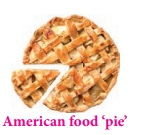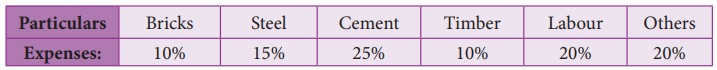Home | | Maths 8th Std | Graphical Representation of the Frequency Distribution for Ungrouped Data

# Graphical Representation of the Frequency Distribution for Ungrouped Data

A graphical representation is the geometrical image of a set of data. It is a mathematical picture. It enables us to think about a statistical problem in visual terms.

Graphical Representation of the Frequency Distribution for Ungrouped Data

A graphical representation is the geometrical image of a set of data. It is a mathematical picture. It enables us to think about a statistical problem in visual terms. A picture is said to be more effective than words for describing a particular thing. The graphical representation of data is more effective for understanding. In the previous classes, we have studied some graphical representations of ungrouped data such as Line graph, Bar graph, and Pictograph. Now, we are going to represent the given ungrouped data in the circular form namely the pie diagram or the pie chart.

1. Pie chart (or) Pie diagram

A pie chart is a circular graph which shows the total value with its components. The circle is divided into sectors and the area of the sectors is proportional to the information given. The area of a circle represents the total value and the different sectors of the circle represent the different components. In the ‘pie chart’ the data are mostly expressed in percentage. Each component is expressed as percentage of the total value.The Pie diagram is so called because the entire graph looks like an American food ‘pie’ and the components like slices cut from ‘pie’.

2. Method of constructing a pie chart:

In a pie chart, we know that the various components are represented by the sectors of a circle and the whole circle represents the sum of the value of all the components. Therefore, the total angle of 360° at the centre of the circle is divided into different sectors according to the value of the components.Sometimes, the value of the components are expressed in percentage. In such cases,Steps for construction of the pie chart:

(i) Calculate the central angle for each component using the above formula and tabulate it.

(ii) Draw a circle of convenient radius and mark one horizontal radius in it.

(iii) Draw radius making central angle of first component with horizontal radius. This sector represents the first component. From this radius, draw next radius with central angle of the second component and so on, until the completion of all components.

(iv) For identification of each sector, shade with different colours.

(v) Label each sector.

Here are given some examples, let us draw the pie chart for the given data.

Example 6.4

Draw a pie diagram to represent the following data, which shows the expenditure of paddy cultivation in 2 acres of land.Also, 1. Find the percentage of the head in which more money had been spent?

2. What percentage of money was spent for seeds?

Solution:

Expenditure of paddy cultivation in 2 acres.1. More money had been spent for wages ₹10,000. Converting into percentage, We have Wages = [10000/36000] ×100% = 27.7%

2. ₹2000 was spent for seeds. Converting into percentage, We have,

Seeds = [2000/36000] ×100% = 5.55%

Example 6.5

Draw a suitable pie chart for the following data relating to the cost of construction of a house.Also, find how much was spent on labour if 55000 was spent for cement.

Solution:

Cost of construction of a house.If the expenses on cement is 55000 then, it represents 25 % and he spent 20 % on labour

Therefore, the expense on Labour = 20/25 × 55000= ₹ 44,000

Note

Uses of pie chart:

1. Pie charts are widely used by the business and the media people.

2. With the help of Pie charts, one can show how the expenditure of the Government or Industry is distributed over different heads.

3. Research people use these type of charts to show their results.

Tags : Statistics | Chapter 6 | 8th Maths , 8th Maths : Chapter 6 : Statistics
Study Material, Lecturing Notes, Assignment, Reference, Wiki description explanation, brief detail
8th Maths : Chapter 6 : Statistics : Graphical Representation of the Frequency Distribution for Ungrouped Data | Statistics | Chapter 6 | 8th Maths